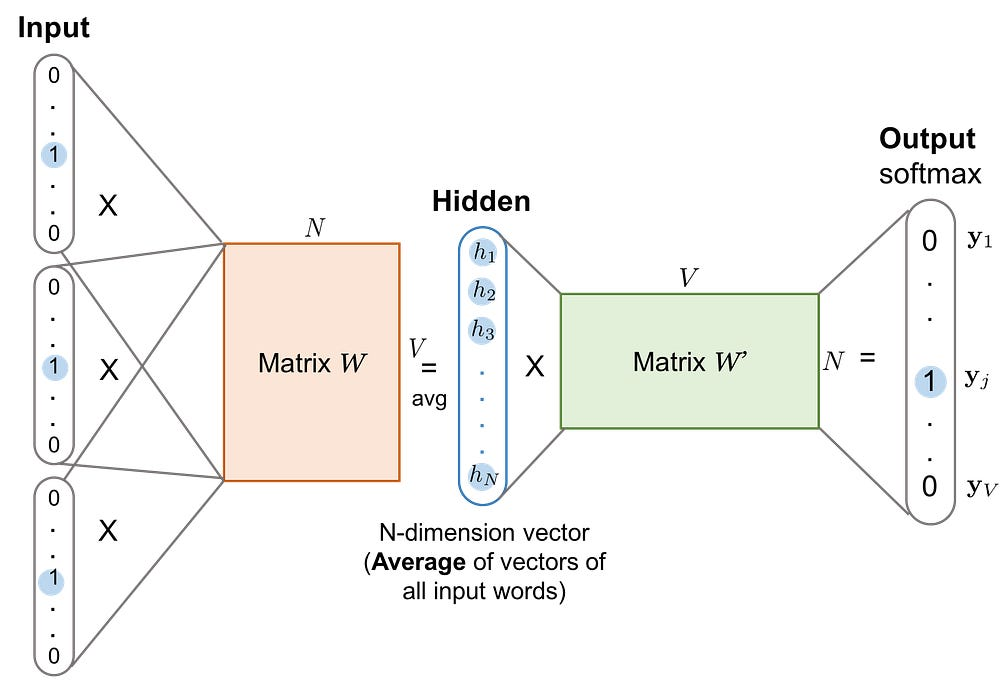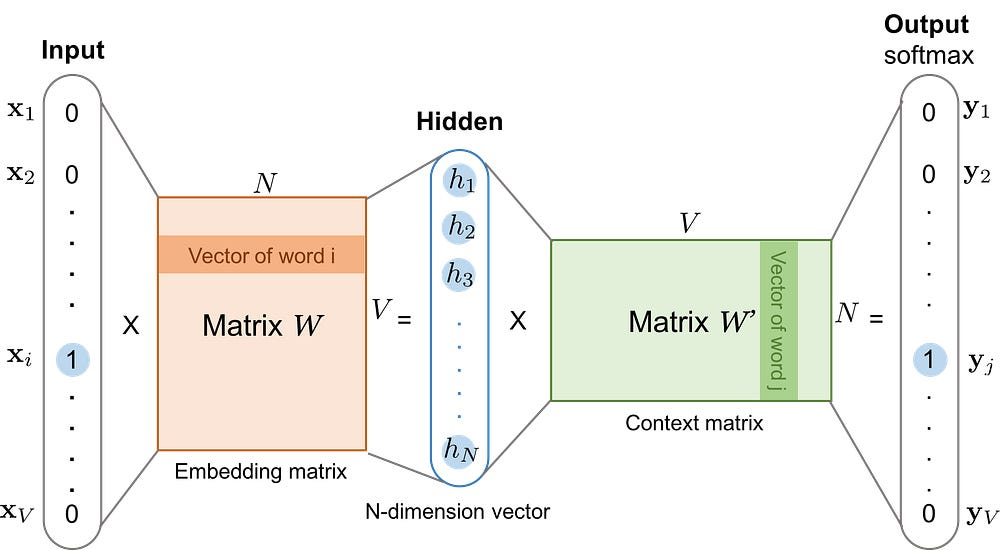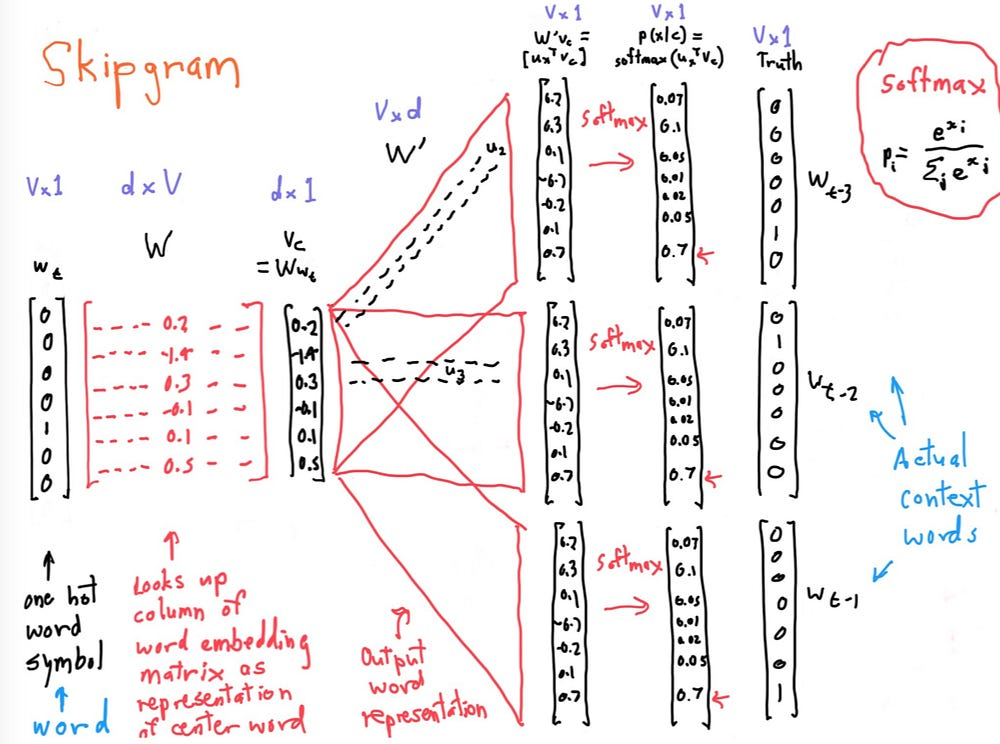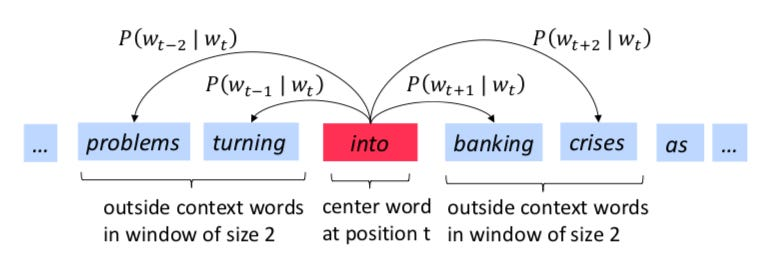# NLP from Zero to One: for classification and machine translation: Part One

Source: Deep Learning on Medium

## Embedding, Word2Vec, Glove, LSTM, Seq2Seq, Attention

### What does NLP do?

We can use NLP to create systems like speech recognition, document summarization, machine translation, spam detection, named entity recognition, question answering, autocomplete, predictive typing and so on.

### What is word Embedding?

Human language (vocabulary) comes in free text. In order to make a machine learning model understand and process the natural language, we need to transform the free-text words into numeric values.

One of the simplest approaches is One-hot encoding.

However, one-hot encoding is impractical computationally:

1. It highly spare (waste large RAM)

2. The multiplication is ZERO, cannot find out the relationship between two one-hot encodings

So, Word embedding represents words and phrases in vectors of non-binary numeric values with much lower and thus denser dimensions. It can be learned using different language models. The word embedding representation is able to reveal hidden relationships. For example, vector(‘Pairs’) — vector(‘France’) == vector(‘Beijing’) — vector(‘China’)

The values in the embedding vector are learned from text and ware based on the surrounding words, as similar words may appear in similar context often.

Two popular examples of embeddings are: Word2Vec and Glove. Two popular methods to train the embeddings are bag-of-words and skip-gram. Please refer to the Stanford Natural Language Processing course for details, which is the best course that I found. http://web.stanford.edu/class/cs224n/

### How to obtain word embedding?

There are two main approaches to learn (train) word embeddings:

1. CBOW: Count-based: unsupervised, matrix factorization of a global word co-occurrence matrix.

2. Skip-gram: Context-based: Supervised. Given a center word, the model will try to predict the surrounding words (yes, reverse to CBOW)

Count based method usually uses TF-IDF vectorization to weight the importance of each word. TF is term-frequency while TF-IDF is term-frequency times inverse document-frequency. Co-occurrence matrix describes how words occur together which shows the relationships between words. PCA is often used to reduce the dimension of co-occurrence matrix.

The overall structure is to learn the weight matrix (W’) between hidden layer and output layer as the word vector representation of the words, where each column represents a word vector.Fig 1. The architecture of CBOW (source:https://lilianweng.github.io/lil-log/2017/10/15/learning-word-embedding.html)

Skip-gram model reverse the use of target and context words, which takes a word to predict surrounding words in a sliding window moving along the sentence. Figure 2 is the architecture of skip-gram. The objective is to learn the weight matrix(W: VXN)between input layer and hidden layer.Fig 2. The architecture of Skip-gram (source:https://lilianweng.github.io/lil-log/2017/10/15/learning-word-embedding.html)

There is a very good picture from Stanford NLP course (Fig. 3), explaining the whole process of skip-gram. The matrix W is the word embedding, with each column representing the embedding for each word. This is just like a two-lay neural network (one linear hidden layer and a output softmax layer). When training this network (determining the embedding and contact word matrices), we will use word pairs. The input will be fed in to this network as a one-hot vector, and the output is also a one-hot vector representing the output word. Then cross-entropy can be used as a loss function between predicted and true one-hot vector.Skip-gram process (source: http://web.stanford.edu/class/cs224n/)

### Word2Vec

After we know what word embedding is and how to obtain the word embeddings, it is quite straightforward to understand Word2Vec. Word2Vec is a framework for learning word embedding vectors. It uses CBOW or skip-gram neural network architecture that we dicussed above. The main idea of word2vec is:

1. go through each word of the whole corpus
2. predict surrounding words of each word: computing P(Wt+j | Wt)learning surrounding words in Word2Vec(source: http://web.stanford.edu/class/cs224n/)

I do not want to go too detail (I may not quite able to do that either 😆). You can find more from google Word2Vec project.

For Word2vec application, I just need to load the word2vec matrix as the first embedding layer and adding other layers (Convolution, LSTM, etc.) on top of that. I will explain it later using examples. There also is a very good example of show word embeddings using Word2Vec (gensim) for the text from Game of Thrones Corpus.

### Glove

The objective of glove is the same as Word2Vec. In word2Vec, we will need to go through all the corpus and predict the surrounding words of each word, which essentially captures co-occurrence of words on at a time. This process is quite computationally intense. “Why not just capture the overall co-occurrence counts directly at one time?”. Then this is the basic concept of Glove. We collect the co-occurrence matrix first, and then use reduce the dimension of this co-occurrence using SVD. Therefore, Glove is combination of count-based matrix factorization and context-based skip-gram model. You can also find more details from Stanford Glove project.

My application of Glove is also similar to that of Word2Vec, using it as a typical “transfer learning” strategy.

### Examples and demos

I will start from a very simple text classification example. We have 13 very simple doc, with “pos” or “neg” labels:

`# define documentsdocs = ['Well done!',        'Good work',        'Great effort',        'nice work',        'Excellent!',        'Weak',        'Poor effort!',        'not good',        'poor work',        'Could have done better.',         'Very Good',         'can be better',         'very poor']`
`# define class labelslabels = array(['pos','pos','pos','pos','pos','neg','neg',               'neg','neg','neg','pos','neg','neg'])`

As discussed above, we need to convert the texts to numerical vectors before processing. Here I used Tokenizer from “keras.preprocessing” :

`# integer encode the documentsvocab_size = 50batch_size = 10num_labels = 2`
`tokenize_train = text.Tokenizer(num_words=vocab_size,                                    char_level=False)`
`tokenize_train.fit_on_texts(docs) `

Let’s have a peep of the fitted results of tokenize_train using

`tokenize_train.word_index`

The output will be:

`{'be': 17,  'better': 6,  'can': 16,  'could': 14,  'done': 4,  'effort': 5,  'excellent': 11,  'good': 1,  'great': 9,  'have': 15,  'nice': 10,  'not': 13,  'poor': 3,  'very': 7,  'weak': 12,  'well': 8,  'work': 2}`

Therefore, tokenizer will use indexes to represent each word in the documents, for example: 2 for “work”, 3 for “poor”, etc. After this preprocess, we can generate the embedding vector for each training doc and labels:

`x_train = tokenize_train.texts_to_matrix(docs, mode='tfidf')`
`encoder = LabelBinarizer()encoder.fit(labels)`
`y_train = encoder.transform(labels)`

So here x_train for each doc will be a 50X1 vector (50 is the vocabulary size). for example x_train is:

`([0.        , 1.44691898, 1.44691898, 0.        , 0.        ,        0.        , 0.        , 0.        , 0.        , 0.        ,        0.        , 0.        , 0.        , 0.        , 0.        ,        0.        , 0.        , 0.        , 0.        , 0.        ,        0.        , 0.        , 0.        , 0.        , 0.        ,        0.        , 0.        , 0.        , 0.        , 0.        ,        0.        , 0.        , 0.        , 0.        , 0.        ,        0.        , 0.        , 0.        , 0.        , 0.        ,        0.        , 0.        , 0.        , 0.        , 0.        ,        0.        , 0.        , 0.        , 0.        , 0.        ])`

Now, let’s develop the neural network for text classification:

`model = Sequential()model.add(Dense(24, input_shape=(vocab_size,)))#FC model.add(Activation('relu'))model.add(Dropout(0.5))model.add(Dense(1))model.add(Activation('sigmoid'))model.summary()`
`model.compile(loss='binary_crossentropy',              optimizer='adam',              metrics=['accuracy'])history = model.fit(x_train, y_train,                    batch_size=batch_size,                    epochs=3,                    verbose=1,                    validation_split=0.1)`

So this is a very simple network, with a fully connect layer with “relu” activation, a dropout layer, followed by an output layer with “sigmoid” activation (as we only have two classes). This fake problem is too simple, so the accuracy is 100%:

`Layer (type)                 Output Shape              Param #    ================================================================= dense_13 (Dense)             (None, 24)                1224       _________________________________________________________________ activation_11 (Activation)   (None, 24)                0          _________________________________________________________________ dropout_6 (Dropout)          (None, 24)                0          _________________________________________________________________ dense_14 (Dense)             (None, 1)                 25         _________________________________________________________________ activation_12 (Activation)   (None, 1)                 0          ================================================================= Total params: 1,249 Trainable params: 1,249 Non-trainable params: 0 _________________________________________________________________ `
`Train on 11 samples, validate on 2 samples Epoch 1/3 11/11 [==============================] - 2s 216ms/step - loss: 0.6297 - acc: 0.6364 - val_loss: 0.5686 - val_acc: 1.0000 `
`Epoch 2/3 11/11 [==============================] - 0s 2ms/step - loss: 0.7208 - acc: 0.7273 - val_loss: 0.5665 - val_acc: 1.0000 `
`Epoch 3/3 11/11 [==============================] - 0s 2ms/step - loss: 0.8326 - acc: 0.4545 - val_loss: 0.5624 - val_acc: 1.0000`

Please see the complete notebooks in GitHub.

### IMDB movie review classification

First, I downloaded the Cornell movie review datasets:

`!wget http://www.cs.cornell.edu/people/pabo/movie-review-data/review_polarity.tar.gz`
`!tar -xvzf review_polarity.tar.gz`

As the texts are raw texts from this datasets, we will need to clean the dataset first, such as removing stop words, removing punctuation:

`def clean_doc(doc): tokens = doc.split() table = str.maketrans('', '', punctuation) tokens = [w.translate(table) for w in tokens] tokens = [word for word in tokens if word.isalpha()] stop_words = set(stopwords.words('english')) tokens = [w for w in tokens if not w in stop_words] tokens = [word for word in tokens if len(word) > 1]return tokens`

The most frequent words are:

`('film', 7983), ('one', 4946), ('movie', 4826), ('like', 3201) ...`

Similarly, we also need to tokenize all the words:

`# load all training reviewstrain_positive_docs = process_docs('txt_sentoken/pos', vocab, True)train_negative_docs = process_docs('txt_sentoken/neg', vocab, True)train_docs = train_negative_docs + train_positive_docs`
`# create the tokenizertokenizer = Tokenizer()tokenizer.fit_on_texts(train_docs)test_encoded_docs = tokenizer.texts_to_sequences(train_docs)        max_length = max([len(s.split()) for s in train_docs])`
`Xtrain = pad_sequences(test_encoded_docs, maxlen=max_length,         padding='post')`
`ytrain = array([0 for _ in range(len(train_negative_docs))] + [1 for         _ in range(len(train_positive_docs))])`

And then develop neural networks:

`vocab_size = len(tokenizer.word_index) + 1`
`# define modelmodel = Sequential()model.add(Embedding(vocab_size, 100, input_length=max_length))model.add(Conv1D(filters=32, kernel_size=8, activation='relu'))model.add(Dropout(0.4))model.add(MaxPooling1D(pool_size=2))model.add(Dropout(0.4))model.add(Flatten())model.add(Dense(10, activation='relu'))model.add(Dense(1, activation='sigmoid'))model.summary()`
`model.compile(loss='binary_crossentropy', optimizer='adam', metrics=['accuracy'])`
`model.fit(Xtrain, ytrain, epochs=5, verbose=2)`
`# evaluateloss, acc = model.evaluate(Xtest, ytest, verbose=0)`
`print('Test Accuracy: %f' % (acc*100))`

Here I used a Conv1D (one dimension convolution layer) to capture the meanings of multiple words together, a MaxPooling layer, a fully connected layer with 10 outputs, and a output layer. One of the best thing in Keras is that we only need to specify the output dimension of each layer. Keras itself will figure out the input dimension.

`_________________________________________________________________ `
`Epoch 1/5  - 1s - loss: 0.6911 - acc: 0.5239 Epoch 2/5  - 1s - loss: 0.6484 - acc: 0.6539 Epoch 3/5  - 1s - loss: 0.4498 - acc: 0.8606 Epoch 4/5  - 1s - loss: 0.1113 - acc: 0.9744 Epoch 5/5  - 1s - loss: 0.0240 - acc: 0.9978 `
`Test Accuracy: 82.000000`

The testing accuracy is 82%.

### Try Word2Vec and Glove

The detailed codes are also in my GitHub repo. I used the Word2Vec model from gensim.

`import gzipimport gensim import re`
`def listlize(review_docs):  for i, line in enumerate(review_docs):    yield gensim.utils.simple_preprocess (line)`
`train_document = list(listlize(train_docs))`
`model_w2v = gensim.models.Word2Vec (train_document, size=150,             window=10, min_count=2, workers=10)model_w2v.train(train_document,total_examples=len(train_document),              epochs=10)`

So using the codes above, we constructed a word2vec model, called model_w2v. In this model, each word is represented by a 1X150 vector, for example:

`print(model_w2v.wv['good'])`
`[ 1.6845648e+00  2.3522677e+00 -8.8309914e-01  5.8164716e-01  -2.6454490e-01  3.3199666e+00 -1.9562098e+00 -2.0406966e+00... -3.5849300e-01 -1.4502057e-01  2.8469378e-01  3.1223300e-01   1.9219459e+00 -5.2754802e-01]`

we can also check the similar words of each word in the vocabulary, for example, the similar words of “restaurant” are: bar, hostage, attendant, etc.

`model_w2v.wv.most_similar ("restaurant")`
`[('bar', 0.9198663830757141),  ('attendant', 0.9040890336036682),  ('trunk', 0.9040547013282776),  ('hostage', 0.8998851180076599),  ('annabel', 0.8998188972473145),  ('deserted', 0.8997957706451416),  ('cisco', 0.8978689908981323),  ('hospital', 0.8939915895462036),  ('flees', 0.8907178640365601),  ('tracks', 0.8899044990539551)]`

After we got the embedding matrix, I am ready to develop the neural works:

`embedding_layer = Embedding(vocab_size, 150, weights=[embedding_vectors], input_length=max_length, trainable=False)model = Sequential()model.add(embedding_layer)model.add(Dropout(0.2))model.add(Conv1D(filters=128, kernel_size=5, activation='relu'))model.add(MaxPooling1D(pool_size=2))model.add(Dropout(0.2))model.add(Flatten())model.add(Dense(1, activation='sigmoid'))`
`model.summary()# compile networkmodel.compile(loss='binary_crossentropy', optimizer='adam', metrics=   ['accuracy'])# fit networkmodel.fit(Xtrain, ytrain, epochs=10, verbose=2)`
`# evaluateloss, acc = model.evaluate(Xtest, ytest, verbose=0)print('Test Accuracy: %f' % (acc*100))`

The accuracy of this model is super low. I have tried to figure it out myself, I still do not know the reason. So I will keep work on improving the accuracy of this work. At the same time, I also tried to use Glove for the same task.

### Try Glove embedding

First of all, I downloaded the glove embedding from Stanford. There are multiple version glove embedding metrics. I downloaded the “6B” one.

`!wget http://nlp.stanford.edu/data/glove.6B.zip!unzip glove*.zip`

Then I used the same procedure as Word2Vec application, creating embedding vectors as the first layer of the neural network:

`# load embedding from fileraw_embedding = load_embedding('glove.6B.100d.txt')embedding_vectors = get_weight_matrix(raw_embedding,    tokenizer.word_index)embedding_layer = Embedding(vocab_size, 100, weights=[embedding_vectors], input_length=max_length, trainable=False)# define modelmodel = Sequential()model.add(embedding_layer)model.add(Dropout(0.2))model.add(Conv1D(filters=128, kernel_size=5, activation='relu'))model.add(MaxPooling1D(pool_size=2))model.add(Dropout(0.2))model.add(Flatten())model.add(Dense(1, activation='sigmoid'))`
`model.summary()`
`# compile networkmodel.compile(loss='binary_crossentropy', optimizer='adam', metrics=  ['accuracy'])model.fit(Xtrain, ytrain, epochs=10, verbose=2)`
`# evaluateloss, acc = model.evaluate(Xtest, ytest, verbose=0)print('Test Accuracy: %f' % (acc*100))`

The accuracy is about 75% in the testing datasets:

`_________________________________________________________________ Epoch 1/10  - 22s - loss: 0.8724 - acc: 0.5122 Epoch 2/10  - 21s - loss: 0.6269 - acc: 0.6794 Epoch 3/10  - 21s - loss: 0.4803 - acc: 0.7978 Epoch 4/10  - 21s - loss: 0.3122 - acc: 0.8989 Epoch 5/10  - 21s - loss: 0.1784 - acc: 0.9611 Epoch 6/10  - 21s - loss: 0.1247 - acc: 0.9683 Epoch 7/10  - 21s - loss: 0.0704 - acc: 0.9911 Epoch 8/10  - 21s - loss: 0.0487 - acc: 0.9956 Epoch 9/10  - 21s - loss: 0.0602 - acc: 0.9900 Epoch 10/10  - 21s - loss: 0.0342 - acc: 0.9967 `
`Test Accuracy: 75.000000`

Just have peep of a review and its prediction:

`print (model.predict_classes(Xtest))print (ytest)`
` 0`

This case (the second review) is predicted correctly 😆 😆

### Multi-class classification

On the examples above, we talked about the binary classification. In this section, I will show a multi-class classification problem in text classification. Here, I got the data for stack overflow topics and comments. I will use the comments to predict the topic tags, such as C#, C++, sql, java, python, etc.

`!wget https://storage.googleapis.com/tensorflow-workshop-examples/stack-overflow-data.csv`
`stack_df = pd.read_csv('stack-overflow-data.csv')`

Same thing, I will need to

1. pre-process the raw text, such as removing stop words, punctuation, and changing numbers with a ‘NUMNUMNUM’ token.
2. Tokenize the text
3. Developing the neural networks

The first network that I used is basic natural network: a fully connected layer with “relu” activation, followed by a dropout layer, and a output layer (with ‘num_labels’ outputs) with ‘softmax’ activation. This is a multi-class classification problem, so ‘softmax’ and ‘categorical_crossentropy’ are used here for output layer and loss.

`model = Sequential()model.add(Dense(512, input_shape=(vocab_size,)))model.add(Activation('relu'))model.add(Dropout(0.5))model.add(Dense(num_labels))model.add(Activation('softmax'))model.summary()`
`model.compile(loss='categorical_crossentropy',              optimizer='adam',              metrics=['accuracy'])history = model.fit(x_train, y_train,                    batch_size=batch_size,                    epochs=3,                    verbose=1,                    validation_split=0.1)`

The accuracy is about 80% after 3 epochs, and the test accuracy is about 79.7%. Let’s take a look at the confusion matrix for the testing results:

We can see 48 ‘iso’ cases are predicted as ‘iphone’ cases, which is reasonable and forgivable (right? 😆), 60 ‘mysql’ cases are predicted as ‘sql’ cases, which is also forgivable.

I also tried adding embedding layer, convolution layer, and LSTM in the neural networks:

`model_conv = Sequential()model_conv.add(Embedding(vocab_size, 100, input_length=100))model_conv.add(Dropout(0.5))model_conv.add(Conv1D(64, 5, activation='relu'))model_conv.add(MaxPooling1D(pool_size=4))model_conv.add(LSTM(100))model_conv.add(Dropout(0.5))model_conv.add(Dense(num_labels, activation='sigmoid'))model_conv.compile(loss='binary_crossentropy', optimizer='adam', metrics=['accuracy'])model_conv.summary()`
`model_conv.compile(loss='categorical_crossentropy',              optimizer='adam',              metrics=['accuracy'])history = model_conv.fit(text_train_sequence, y_train,                    batch_size=batch_size,                    epochs=10,                    verbose=1,                    validation_split=0.1)`

The validation accuracy is about 72.4% and the testing accuracy is about 73.3%. The accuracy is lower than that from the basic neural networks, but we can see there is no “overfitting” due to the dropout layers 😆. The complete notebook are also in my git repo: Stack_topic_Classification.ipynb.

I intended to write everything, including everything here as well as details about LSTM, seq2seq for machine translation, in a single blog. But this blog is very long already. I will have a “part two” blog for other parts. Please check it back later. Thank you 😆.

I am learning deep learning. If you have any suggestions regarding my work and my study, please let me know. Thank you very much.